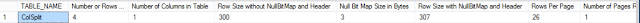## Scenario:

You are working as SQL Server DBA or Developer, You need to create a table with some columns of different data types. You need to estimate the storage how much space this table will take with some estimated records.

The below script can be used to get the estimated storage from the table/s you like. In first query , you will provide the table name or tables name with number of rows you would like to get estimated storage.

## Solution:

The script below can be used to estimate the size of tables in SQL Server Database with number of rows we want to estimate.
```/*----------------------------------------
1:Build the List of Tables with Rows for which Estimation have to be done
------------------------------------------*/
;
WITH CTE_TableNames
AS (SELECT 'TableName1' AS TableName,
4         AS Num_Rows
UNION ALL
SELECT 'TableName1' AS TableName,
200         AS Num_Rows
)
/*----------------------------------------
2:Get Storage Size for Fixed Column and Variable Columns
------------------------------------------*/
,
CTE_DataTypes
AS (SELECT TABLE_NAME,
TABLE_SCHEMA,
COLUMN_NAME,
IS_NULLABLE,
DATA_TYPE,
CHARACTER_MAXIMUM_LENGTH,
CASE
WHEN Data_Type = 'Int' THEN 4
WHEN DATA_TYPE = 'BigInt' THEN 8
WHEN DATA_TYPE = 'TinyInt' THEN 1
WHEN DATA_TYPE = 'SmallInt' THEN 2
WHEN DATA_TYPE = 'Bit' THEN 1
WHEN DATA_TYPE = 'Money' THEN 8
WHEN DATA_TYPE = 'SmallMoney' THEN 4
WHEN DATA_TYPE = 'SmallDateTime' THEN 4
WHEN DATA_TYPE = 'DateTime' THEN 8
WHEN DATA_TYPE = 'Date' THEN 3
WHEN DATA_TYPE = 'Real' THEN 4
WHEN DATA_TYPE = 'Float'
AND NUMERIC_PRECISION <= 24 THEN 4
WHEN DATA_TYPE = 'Float'
AND NUMERIC_PRECISION >= 25 THEN 8
WHEN DATA_TYPE IN ( 'Decimal', 'Numeric' )
AND NUMERIC_PRECISION <= 9 THEN 5
WHEN DATA_TYPE IN ( 'Decimal', 'Numeric' )
AND NUMERIC_PRECISION BETWEEN 10 AND 19 THEN 9
WHEN DATA_TYPE IN ( 'Decimal', 'Numeric' )
AND NUMERIC_PRECISION BETWEEN 20 AND 28 THEN 13
WHEN DATA_TYPE IN ( 'Decimal', 'Numeric' )
AND NUMERIC_PRECISION BETWEEN 29 AND 38 THEN 17
WHEN DATA_TYPE = 'Time'
AND DATETIME_PRECISION IN ( 0, 1, 2 ) THEN 3
WHEN DATA_TYPE = 'Time'
AND DATETIME_PRECISION IN ( 3, 4 ) THEN 4
WHEN DATA_TYPE = 'Time'
AND DATETIME_PRECISION >= 5 THEN 5
WHEN DATA_TYPE = 'DateTime2'
AND DATETIME_PRECISION < 3 THEN 6
WHEN DATA_TYPE = 'DateTime2'
AND DATETIME_PRECISION IN( 3, 4 ) THEN 7
WHEN DATA_TYPE = 'DateTime2'
AND DATETIME_PRECISION > 4 THEN 8
WHEN DATA_TYPE = 'DateTimeoffset'
AND DATETIME_PRECISION < 3 THEN 8
WHEN DATA_TYPE = 'DateTimeoffset'
AND DATETIME_PRECISION IN( 3, 4 ) THEN 9
WHEN DATA_TYPE = 'DateTimeoffset'
AND DATETIME_PRECISION > 4 THEN 10
ELSE CHARACTER_OCTET_LENGTH --This is going to cover CHAR,NCHAR,VARCHAR,NVARCHAR Columns
END AS SIZE_IN_BYTES
FROM   INFORMATION_SCHEMA.COLUMNS),
CTE_Num_Cols
AS (SELECT TD.Table_NAME,
Count(TD.COLUMN_NAME) Num_Cols,
Sum(SIZE_IN_BYTES)    AS SIZE_IN_BYTES,
TN.Num_Rows
FROM   CTE_DataTypes TD
INNER JOIN CTE_TableNames TN
ON TD.TABLE_NAME = TN.TableName
GROUP  BY TD.Table_NAME,
TN.Num_Rows),
NullBitMap_RowSize
AS (SELECT Table_Name,
Num_Rows,
Num_Cols,
Size_In_Bytes,
2 + ( ( Num_Cols + 7 ) / 8 )                         AS NullBitMap,
Size_In_Bytes + ( 2 + ( ( Num_Cols + 7 ) / 8 ) ) + 4 AS Row_Size
FROM   CTE_Num_Cols),
Rows_Per_Page
AS (SELECT TABLE_NAME,
Num_Rows,
Num_Cols,
SIZE_IN_BYTES,
NullBitMap,
Row_Size,
8096 / ( Row_Size + 2 ) AS Rows_Per_Page
FROM   NullBitMap_RowSize),
Num_Pages
AS (SELECT TABLE_NAME,
Num_Rows,
Num_Cols,
SIZE_IN_BYTES,
NullBitMap,
Row_Size,
Rows_Per_Page,
Ceiling(Num_Rows / Cast(Rows_Per_Page AS NUMERIC(30, 1))) AS Num_Pages
FROM   Rows_Per_Page)
SELECT TABLE_NAME,
Num_Rows                             AS [Number or Rows for Estimation],
Num_Cols                             AS [Number of Columns in Table],
SIZE_IN_BYTES                        AS [Row Size without NullBitMap and Header],
NullBitMap                           AS [Null Bit Map Size in Bytes],
Row_Size                             AS [Row Size with NullBitMap and Header],
Rows_Per_Page                        AS [Rows Per Page],
Num_Pages                   AS [Number of Pages Required to Store Given Rows],
8192 * Num_Pages                     AS Heap_Size_in_Bytes,
Ceiling(( 8192 * Num_Pages ) / 1024) AS Heap_Size_in_KB
FROM   Num_Pages```How to get estimated Size of Table in SQL Server Table for Future Planning